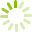# Foundations of Mathematics 11 Part 2 (MATH 0872)

Students will solve problems in geometry, measurement, trigonometry and right angle triangles, statistics and financial math. Students will be able to look for patterns, make inferences, draw scale diagrams of 2-D and 3-D shapes, simplify problems, eliminate possibilities and develop alternative approaches to mathematical problems. Students will use a calculator to evaluate complex expressions using special keys to perform a variety of functions.

Both MATH 0862 and MATH 0872 are required for ABE Advanced Level Foundations of Mathematics.

The use of a graphing calculator or other technology is optional.
Course code: MATH 0872
Credits: 4.0
Length: 96.0 hours
Course outline: view https://www.vcc.ca/vccphp/courseoutline?subject=MATH&number=0872

## Prerequisites

VCC MATH 0862 with a C-; or 52 % on the VCC Intermediate Algebra Assessment.